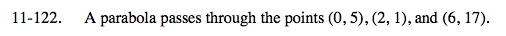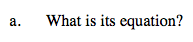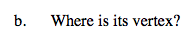### Home > A2C > Chapter Ch11 > Lesson 11.2.6 > Problem11-122

11-122.Create a system of equations based on the general equation
y = ax2 + bx + c:
(5) = a(0)2 + b(0) + c
(1) = a(2)2 + b(2) + c
(17) = a(6)2 + b(6) + c

Simplify and solve.
5 = c
1 = 4a + 2b + c
17 = 36a + 6b + c

y = x2 − 4x + 5Convert the equation you found in part (a) into graphing form.